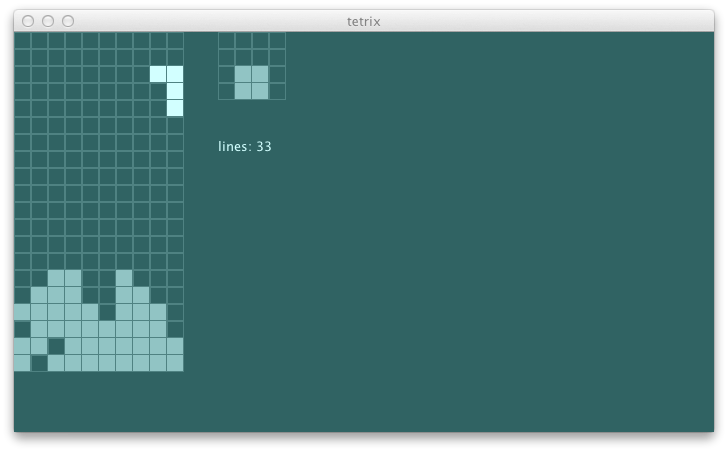### other parameters

This got me thinking what if I change the balance between other penalties like height?

``````    val heightWeight = 2
val weightedHeights = heights.values map {heightWeight * _}
``````

Here are the results:

``````h1:c3 = lines: Vector(9, 16, 8, 15, 12)   // 12 +/- 4
h2:c3 = lines: Vector(13, 19, 9, 16, 12)  // 13 +/- 6
h3:c3 = lines: Vector(20, 20, 20, 18, 43) // 20 +/- 23
h4:c3 = lines: Vector(26, 39, 11, 22, 35) // 26 +/- 13
h5:c3 = lines: Vector(22, 25, 11, 19, 16) // 19 +/- 8
``````

This is 20 +/- 23 lines and 26 +/- 13! With h4 both the min and the max performer has degraded, but the median has increased. I like h3 because it has the largest minimum.

The only parameter we haven’t tested now is `coverupsWeight`. We’ll denote this as `v1`, `v2`, etc:

``````h3:c3:v1 = lines: Vector(20, 20, 20, 18, 43) // 20 +/- 23
h3:c3:v2 = lines: Vector(11, 13, 12, 14, 17) // 13 +/- 4
``````

This is 13 +/- 4 lines, so clearly not a good idea. How about we eliminate it from penalty altogether?

``````h3:c3:v0 = lines: Vector(35, 34, 22, 27, 33) // 33 +/- 11
``````

The data does not lie. 33 +/- 11 lines. The cavitiy analysis was useless. Here are the results from tweaking the balance of `heightWeight` and `crevasseWeight`:

``````h0:c1:v0   = lines: Vector(0, 0, 0, 1, 0)      // 0 +/- 1
h1:c2:v0   = lines: Vector(35, 21, 19, 27, 21) // 21 +/- 14
h1:c1:v0   = lines: Vector(35, 34, 22, 27, 33) // 33 +/- 11
h12:c11:v0 = lines: Vector(32, 36, 23, 46, 29) // 32 +/- 14
h11:c10:v0 = lines: Vector(34, 34, 23, 52, 29) // 34 +/- 18
h10:c9:v0  = lines: Vector(31, 34, 23, 50, 29) // 31 +/- 19
h9:c8:v0   = lines: Vector(31, 34, 24, 50, 29) // 31 +/- 19
h8:c7:v0   = lines: Vector(31, 34, 24, 50, 29) // 31 +/- 19
h7:c6:v0   = lines: Vector(31, 26, 25, 50, 29) // 29 +/- 21
h6:c5:v0   = lines: Vector(31, 26, 25, 50, 29) // 29 +/- 21
h5:c4:v0   = lines: Vector(31, 25, 14, 49, 32) // 32 +/- 18
h4:c3:v0   = lines: Vector(31, 37, 13, 44, 27) // 31 +/- 18
h3:c2:v0   = lines: Vector(40, 36, 13, 31, 20) // 31 +/- 18
h2:c1:v0   = lines: Vector(29, 29, 16, 24, 17) // 24 +/- 8
h1:c0:v0   = lines: Vector(8, 6, 8, 11, 8)     // 8 +/- 3
``````

If we choose using median, h11:c10:v0 is the winner. Here’s the modified `penalty`:

``````  def penalty(s: GameState): Double = {
val groupedByX = s.unload(s.currentPiece).blocks map {_.pos} groupBy {_._1}
val heights = groupedByX map { case (k, v) => (k, v.map({_._2 + 1}).max) }
val heightWeight = 11
val weightedHeights = heights.values map {heightWeight * _}
val hWithDefault = heights withDefault { x =>
if (x < 0 || x > s.gridSize._1 - 1) s.gridSize._2
else 0
}
val crevassesWeight = 10
val crevasses = (-1 to s.gridSize._1 - 2) flatMap { x =>
val down = hWithDefault(x + 1) - hWithDefault(x)
val up = hWithDefault(x + 2) - hWithDefault(x + 1)
if (down < -2 && up > 2) Some(math.min(crevassesWeight * hWithDefault(x), crevassesWeight * hWithDefault(x + 2)))
else None
}
math.sqrt((weightedHeights ++ crevasses) map { x => x * x } sum)
}
``````

The good old swing UI still works, and it plays nicely:We’ll continue from here tomorrow. As always, the code is up on github.

``````\$ git fetch origin
\$ git co day10v2 -b try/day10
\$ sbt library/run
\$ sbt swing/run
``````